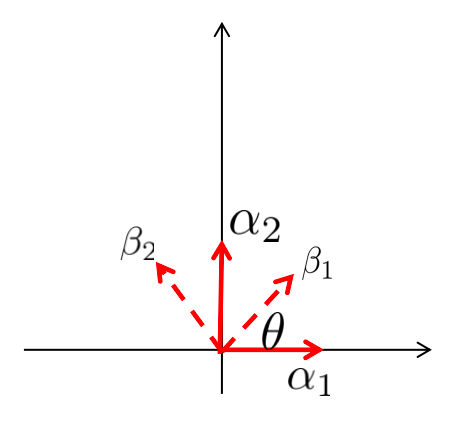# 【数学基础】第十四课：线性代数

## 线性空间，基，线性映射，线性变换，基变换，线性回归

Posted by x-jeff on June 26, 2020

# 1.线性空间与基

⚠️线性空间必须要有原点。例如一个有原点的平面就是一个线性空间（有了原点才能定义向量及其运算）。

‼️是线性空间里的一组线性无关向量，使得任何一个向量都可以唯一的表示成这组基的线性组合。基给出了定量描述线性结构的方法：坐标系。坐标即为基的系数。

# 2.线性映射与矩阵

👉线性映射是从一个向量空间V到另一个向量空间W的映射且保持加法运算和数量乘法运算。线性映射的本质就是保持线性结构的映射。

👉线性变换是线性空间V到其自身的线性映射。

👉线性映射的矩阵描述：

V,W分别为n,m维的线性空间，$\alpha=\{ \alpha_1 , … , \alpha_n \},\beta=\{ \beta_1 , … , \beta_m \}$分别为V,W的一组基。$T:V \to W$是一个线性映射。于是$T,\alpha,\beta$唯一决定一个矩阵$A_{\alpha,\beta}(T)=[A_{ij}]_{m\times n}$，使得：

$T(\alpha_j)=\sum^m_{i=1} A_{ij} * \beta_i , \forall j \in 1,...,n \tag{1}$

$T(\alpha_1,...,\alpha_n)=(\beta_1,...,\beta_m) \cdot A_{\alpha,\beta}(T) \tag{2}$

$T(\alpha)=\beta \cdot A_{\alpha,\beta}(T) \tag{3}$

👉举个例子，假设我们现在有线性空间V，基为$\{ \alpha_1,\alpha_2 \}$。我们将其逆时针旋转$\theta$得到新的线性空间W，基为$\{ \beta_1,\beta_2 \}$（假设基都为单位向量）。那么该如何描述这个旋转映射呢？$T(\beta_1)=\alpha_1 \cos \theta + \alpha_2 \sin \theta = (\alpha_1 , \alpha_2 ) \begin{bmatrix} \cos \theta \\ \sin \theta \\ \end{bmatrix}$ $T(\beta_2)=-\alpha_1 \sin \theta + \alpha_2 \cos \theta = (\alpha_1 , \alpha_2 ) \begin{bmatrix} -\sin \theta \\ \cos \theta \\ \end{bmatrix}$ $T(\beta_1,\beta_2)=(\alpha_1,\alpha_2) \begin{bmatrix} \cos \theta & -\sin \theta \\ \sin \theta & \cos \theta \end{bmatrix} \tag{4}$

👉再举另外一个例子，如果我们要将三维空间（基为$\{ \alpha_1 , \alpha_2 , \alpha_3 \}$）线性映射到二维空间（基为$\{ \beta_1,\beta_2 \}$）,假设有：

• $T(\alpha_1)=\beta_1$
• $T(\alpha_2)=\beta_2$
• $T(\alpha_3)=\beta_1+\beta_2$

$T(\alpha_1,\alpha_2,\alpha_3)=(\beta_1,\beta_2) \begin{bmatrix} 1 & 0 & 1 \\ 0 & 1 & 1 \\ \end{bmatrix}$

👉如果我们选取V,W的另外一组基，$\widetilde{\alpha}=\alpha \cdot P,\widetilde{\beta}=\beta \cdot Q$，那么存在矩阵$A_{\widetilde{\alpha},\widetilde{\beta}}(T)$使得，

$T(\widetilde{\alpha})=\widetilde{\beta} \cdot A_{\widetilde{\alpha},\widetilde{\beta}}(T)$

P,Q为基的变换矩阵。

$\widetilde{\alpha_1}=\alpha_1+2\alpha_2$
$\widetilde{\alpha_2}=3\alpha_1+4\alpha_2$

$(\widetilde{\alpha_1},\widetilde{\alpha_2})=[\alpha_1,\alpha_2] \begin{bmatrix} 1 & 3 \\ 2 & 4 \\ \end{bmatrix}$ $\begin{bmatrix} 1 & 3 \\ 2 & 4 \\ \end{bmatrix}$

$T(\alpha) \cdot P = T(\alpha \cdot P)=\beta \cdot Q \cdot A_{\widetilde{\alpha},\widetilde{\beta}}(T)$

$Q \cdot A_{\widetilde{\alpha},\widetilde{\beta}}(T) \cdot P^{-1}=A_{\alpha,\beta}(T) \tag{5}$

# 3.线性回归

$X \cdot \beta =Y \tag{6}$

👉代数解法：

$X^T X \cdot \beta = X^T Y$ $\beta = (X^T X) ^ {-1} X^T Y \tag{7}$

👉最小二乘法：【机器学习基础】第六课：线性回归

$\begin{bmatrix} A & B \\ C & D \\ \end{bmatrix} ^ {-1} = \frac{1}{AD-BC} \begin{bmatrix} D & -B \\ -C & A \\ \end{bmatrix}$

# 4.参考资料